Share

# Irrational Numbers

Course

#### notes

We saw, in the previous section, that there may be numbers on the number line that are not rationals. In this section, we are going to investigate these numbers. So far, all the numbers you have come across, are of the form p/q  where p and q are integers and q ≠ 0. So, you may ask: are there numbers which are not of this form? There are
indeed such numbers.

The Pythagoreans in Greece, followers of the famous mathematician and philosopher Pythagoras, were the first
to discover the numbers which were not rationals, around 400 BC. These numbers are called irrational numbers
(irrationals), because they cannot be written in the form of a ratio of integers. There are many myths surrounding the
discovery of irrational numbers by the Pythagorean, Hippacus of Croton. In all the myths, Hippacus has an
unfortunate end, either for discovering that √2 is irrational or for disclosing the secret about √2 to people outside the
secret Pythagorean sect!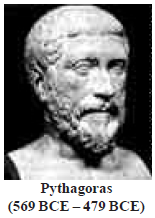Fig. 1.3

Let us formally define these numbers.
A number ‘s’ is called irrational, if it cannot be written in the form p/q, where p and q are integers and q ≠ 0.

You already know that there are infinitely many rationals. It turns out that there are infinitely many irrational numbers too. Some examples are: √2, √3, √15; π, 0.10110111011110...

Remark : Recall that when we use the symbol √ , we assume that it is the positive square root of the number. So √4 = 2, though both 2 and –2 are square roots of 4.

Some of the irrational numbers listed above are familiar to you. For example, you
have already come across many of the square roots listed above and the number p.
The Pythagoreans proved that √2 is irrational. Later in approximately 425 BC,
Theodorus of Cyrene showed that √3, √5, √6, √7, √10, √11, √12, √13, √14, √15 and √17 are also irrationals. Proofs of irrationality of √2, √3, √5, etc., shall be discussed in Class X. As to p, it was known to various cultures for thousands of years, it was proved to be irrational by Lambert and Legendre only in the late 1700s. In the next section, we will discuss why 0.10110111011110... and p are irrational.

Remember the bag of rational numbers. If we now put all irrational numbers into the bag, will there be any number left on the number line? The answer is no! It turns out that the collection of all rational numbers and irrational umbers together make up what we call the collection of real numbers, which is denoted by R. Therefore, a real number is either rational or irrational. So, we can say that every real number is represented by a unique point on the number line. Also, every point on the number line represents a unique real number.
This is why we call the number line, the real number line.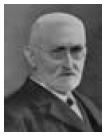R. Dedekind (1831-1916)
Fig. 1.4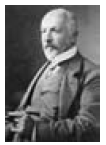G. Cantor (1845-1918)
Fig. 1.5

In the 1870s two German mathematicians, Cantor and Dedekind, showed that : Corresponding to every real number, there is a point on the real number line, and corresponding to every point on the number line, there exists a unique real number.

Let us see how we can locate some of the irrational numbers on the number line.

Example  : Locate √2 on the number line.
Solution : It is easy to see how the Greeks might have discovered √2 . Consider a unit square OABC, with each side 1 unit in length (see Fig. 1.6). Then you can see by the Pythagoras theorem that OB = √12 + √12 = √2 . How do we represent √2 on the number line?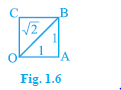This is easy. Transfer Fig. 1.6 onto the number line making sure that the vertex O
coincides with zero (see Fig. 1.7).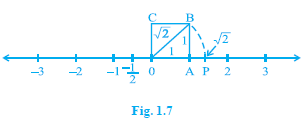We have just seen that OB = 2 . Using a compass with centre O and radius OB, draw an arc intersecting the number line at the point P. Then P corresponds to 2 on the number line.
Example  : Locate 3 on the number line.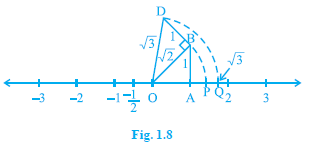Construct BD of unit length perpendicular to OB (as in Fig. 1.8). Then using the Pythagoras theorem, we see that OD = sqrt((sqrt2)^2+1^2) = sqrt3 .Using a compass, with centre O and radius OD, draw an arc which intersects the number line at the point Q. Then Q corresponds to √3.
In the same way, you can locate √n for any positive integer n, after sqrt(n − 1) has been
located.

### Shaalaa.com

Irrational Numbers [00:03:33]
S
0%

S